The changing atom

History of the atom

·        The model of the atom has changed as our observations of its behaviour and properties have increased.

·        A model is used to explain observations.  The model changes to explain any new observations.

·        A map gives you an overview of a town/city.  As technology has improved our maps have become more accurate and detailed.  This is the same for scientific models.

·        Research one of the stages in the development of the atom and present your findings to the group:-

Democritus (5th century)                                  The Greek 'atom' - indivisible

John Dalton (1800's)                                          Atoms of the same element are the same

George Johnstone Stoney (1891)                   Electrolysis.  The charge of an electron.

Joseph J Thompson (1897)                             The cathode ray tube and e/m deflection.  The mass / charge of an electron.

Joseph J Thompson (1906)                             ‘Plum – Pudding’ model of an atom.

Robert Milikan (1909)                                         Oil drop experiment.  The mass / charge of an electron.

Rutherford (and Marsden) (1909)                    Alpha particle deflection.  The nuclear model.

Henry Moseley (1913)                                        Atomic number

Neils Bohr (1913)                                                 Planetary model of the atom

Louis De Broglie (1923)                                     Wave particle duality

Erwin Shrodinger (1926)                                   Atomic orbitals

James Chadwick (1932)                                    Discovery of the neutron

Atomic structure

Protons, electrons and neutrons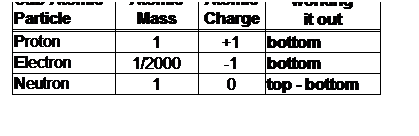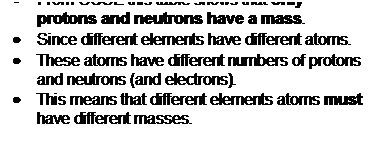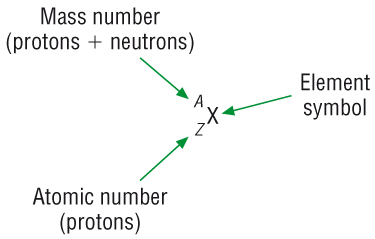·        So an atom of one element must have a different mass from another element, we call this the Mass Number.

·        The number of protons determines which element an atom is and the bottom number tell us this, we call this the Atomic number.

Examples:-

1) Lithium

 7 Li 3

Protons = 3

Electrons = 3

Neutrons = 4  (7-3)

2)  Nitrogen

 14 N 7

Protons = 7

Electrons = 7

Neutrons = 7  (14-7)

Isotopes

An atom of the same element that has the same number of protons and electrons but a different number of neutrons.

• As the atom has the same number of protons and electrons it will have the same chemical properties.
• They are all hydrogen atoms because they all have the same number of protons
• Hydrogen can be used as an example:-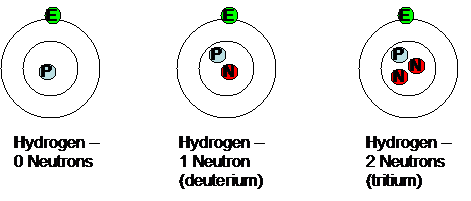Atomic structure of ions

• When an atom becomes an ion it must have gained or lost electrons.

• This will have an effect on its atomic structure:

Sodium ion

• The sodium ion has one less electron than its number of protons therefore the charge is 1+ (as electrons are negative)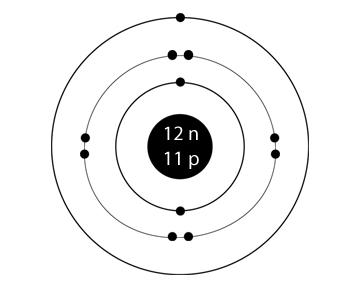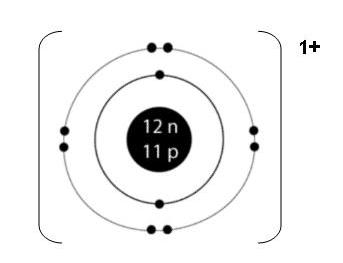Mass number, A = 23 Mass number, A = 23 Atomic number, Z, P = 11  (+) Atomic number, Z, P = 11  (+) Electrons, e = 11  (-) Electrons, e = 10  (-) Neutrons, N = 12 Neutrons, N = 12 charge is neutral, 11P (+) = 11e (-) charge is 1+, 11P (+) = 10e (-)

Chloride ion:

• The chloride ion has one more electron than its number of protons therefore the charge is 1- (as electrons are negative)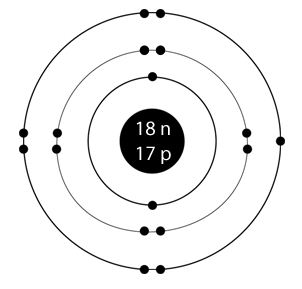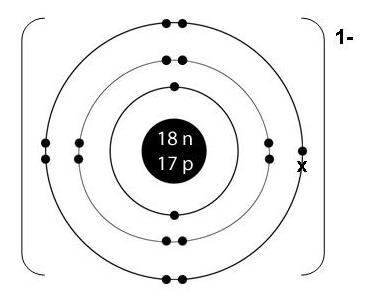Mass number, A = 35 Mass number, A = 35 Atomic number, Z, P = 17  (+) Atomic number, Z, P = 17  (+) Electrons, e = 17  (-) Electrons, e = 18  (-) Neutrons, N = 18 Neutrons, N = 18 charge is neutral, 17P (+) = 17e (-) charge is 1-, 17P (+) = 18e (-)

Questions 1, 2 p7 / 1 p35 / 1p36

Chemical equations:

Writing formulae and balancing equations

·        The first part of this is to be able to write formulae.

·        Some names are straightforward whereas others are unexpected.

Writing formula

·        A formulae is a shorthand way of describing a chemical substance.

·        The composition and elements present are represented in the chemical formulae.

·        Following a set of rules the chemical formula for a substance can deduced:-

 Periodic Table Group Charge on ion Other ions 1 1+ H+  NH4+  Ag+ 2 2+ Co2+  Cu2+  Fe2+ 3 3+ Fe3+ 6 2- SO42-  CO32- 7 1- OH-  NO3-

·         The ions in a chemical formula must add up to zero.

·         Use subscripts after an ion in a formula to double/triple that ion so the sum=0.  eg. CuCl2

·         If you are double/tripling ions that consist of more than one element brackets must be used. eg.  Ca(OH)2

·         If Roman numeral numbers follow a metal ion in brackets, that tells you the positive charge of that metal ion.  They are usually Transition Metals as they can have more than one oxidation state.  eg.  Copper (II) Chloride.  Copper can also exist as copper (I).

·         Water of crystallization can be added to compounds after a dot.  eg.  CuSO4.7H2O

How to work out formulae

Sodium Chloride -            Na+               Cl-                 Write the ions with charges

1          :        1                    Write the ratio of the charges

Scale up if necessary to =0

NaCl                          Bring together omitting the charges

Copper (II) Chloride          Cu2+              Cl-                 Write the ions with charges

2          :        1                    Write the ratio of the charges

Cu2+     :        Cl-2                     Scale up if necessary to =0

CuCl2                                Bring together omitting the charges

Calcium Hydroxide           Ca2+              OH-               Write the ions with charges

2          :        1                    Write the ratio of the charges

Ca2+    :        (OH-)2           Scale up if necessary to =0

Ca(OH)2                   Bring together omitting the charges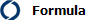Writing simple formula from the periodic tableWriting complex formula

Balancing equations

• One of the most important concepts in chemistry is that mass is always conserved.

• You always have exactly the same at the end as what you started.

• If you follow 4 steps – you cant go wrong:-

Step 1          Write out the word equation.

Iron     +       Hydrochloric acid    à     Iron (II) chloride        +       Hydrogen

Step 2          Write the correct formula underneath.

Iron      +       Hydrochloric acid        à     Iron (II) chloride            +       Hydrogen

Fe        +       HCl                               à     FeCl2                           +       H2

Step 3          Balance using large numbers to scale up the number of molecules.  A rule of thumb to help you balance is to balance the elements in this order - MACHO:

Metal

Any other element

Carbon

Hydrogen

Oxygen

A table helps.

Iron      +       Hydrochloric acid        à     Iron (II) chloride            +       Hydrogen

Fe        +       2HCl                             à     FeCl2                            +       H2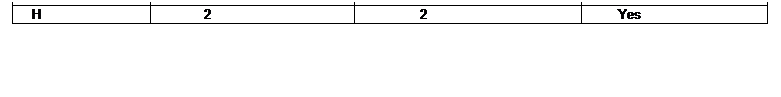Put the 2 before the molecule with the element you are scaling up.  You now have the same number of atoms on each side.

Step 4          All that remains is to add the state symbols.

Iron      +       Hydrochloric acid        à     Iron (II) chloride            +       Hydrogen

Fe        +       2HCl                             à     FeCl2                            +       H2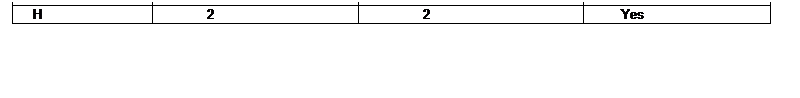Fe(s)     +       2HCl(aq)                        à     FeCl2(aq)                       +       H2(g)

You now have a balanced chemical equation.  This can be used to record an experiment or calculate amounts to mix.

Questions 1-2 p19 / 11 p35

Acids and Bases

• pH less than 7.

• Turn litmus red.

• Is neutralised by alkali and bases.

Strong acids - Hydrochloric acid:

Strong acids dissociate fully in water releases H+ ions into the solution:

• When hydrogen chloride is bubbles through water it dissolves.  With water,  it completely ionises:

HCl(g)                à            H+(aq)             +                          Cl-(aq)

Acids can be defined as proton donors

Weak acids - Ethanoic acid:

Weak acids partially dissociate in water

• Weak acids such as ethanoic acid only dissociate a small amount giving a small amount of hydrogen ions:

CH3COOH(l)        +        aq                D            H+(aq)             +                          CH3COO-(aq)

Bases

• These are chemical opposites to acids which include:  metal oxides, hydroxides, ammonia and amines.

• They neutralise acids.

Bases can be defined as proton acceptors

Alkalis

• These are solutions - when a compound dissolved in water gives a solution with a pH of greater than 7

• Some common alkalis:  sodium hydroxide, potassium hydroxide and ammonia.

• Alkalis are more corrosive than acids - a lower concentration will be more corrosive than the corresponding acid.

• An alkali forms hydroxide ions in aqueous solution:

NaOH(s)        +        aq             à            Na+(aq)             +                          OH-(aq)

Alkalis dissociate to give hydroxide ions in solution

• Weak bases such as ammonia only dissociate a small amount giving a small amount of hydroxide ions:

NH3(g)        +        H2O(l)               D               NH4+(aq)        +        OH-(aq)

Biological acids and bases

Acids:  fatty acids, amino acids, nucleic acids

Amphoteric:  This means they have acidic and basic properties - amino acids - NH2 and CO2H

Questions 1-2 p23 / 12 p35

Salts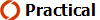(investigative)(Acid / Base - done earlier with moles)

(NOTE:  Alternatively do the practical as a circus cf GCSE)

All salts contain the following:

• A positive cation - usually a metal or ammonium (NH4+)

• A negative anion derived from an acid:

 Acid Cation...Salt Sulphuric ...sulphate H2SO4 ...SO42- Nitric ...nitrate HNO3 ...NO3- Hydrochloric ...chloride HCl ...Cl-
• These salts are formed with one of the following reactions:

1    Metal                                  +    Acid     à      Salt         +    Hydrogen

2    Metal Oxide                       +    Acid     à      Salt         +    Water

3    Metal Hydroxide (alkali)   +    Acid     à      Salt         +    Water

4    Metal Carbonate               +    Acid     à      Salt         +    Water        +       Carbon dioxide

Constructing balanced chemical equations:

• Given 2 reactants it is possible to construct balanced chemical equations:

Example:  Sodium hydroxide reacts with sulphuric acid, write a balanced chemical equation for this reaction:

Step 1          Use the 4 reactions above to identify your reaction - Sodium hydroxide tells us it is reaction (3)

Sodium hydroxide     +       Sulphuric acid    à     Salt        +       Water

Step 2          Work out the name of your salt:

Sodium hydroxide     +       Sulphuric acid    à     Sodium sulphate        +       Water

Step 3          Write the correct formula underneath.

Sodium hydroxide     +       Sulphuric acid    à     Sodium sulphate        +       Water

NaOH                  +            H2SO4           à            Na2SO4                +          H2O

Step 4          Balance the equation, in this order - works 90% of the time

Metal

Any other element

Carbon

Hydrogen

Oxygen

Sodium hydroxide     +       Sulphuric acid    à     Sodium sulphate        +       Water

2NaOH                   +            H2SO4           à            Na2SO4                +          H2O

 Element Balanced Na 1 2 NO S H O

Sodium hydroxide     +       Sulphuric acid    à     Sodium sulphate        +       Water

2NaOH                   +            H2SO4           à            Na2SO4                +        2H2O

 Element Balanced Na 1 x 2 = 2 2 Yes S 1 1 Yes H 4 2 NO O

Sodium hydroxide     +       Sulphuric acid    à     Sodium sulphate        +       Water

2NaOH                   +            H2SO4           à            Na2SO4                +        2H2O

 Element Balanced Na 1 x 2 = 2 2 Yes S 1 1 Yes H 4 2 No O

Sodium hydroxide     +       Sulphuric acid    à     Sodium sulphate        +       Water

2NaOH                   +            H2SO4           à            Na2SO4                +        2H2O

 Element Balanced Na 1 x 2 = 2 2 Yes S 1 1 Yes H 4 2 x 2 = 4 Yes O 6 6 Yes

Step 4          Add the state symbols:

2NaOH(aq)              +         H2SO4(aq)           à          Na2SO4(aq)            +        2H2O(l)

You now have a balanced chemical equation.Acid salts:

• Sulphuric acid has 2 H+ ions, we call these diprotic acids as they can both be replaced:

H2SO4           à          NaHSO4        Sodium hydrogen sulphate

• NaHSO4 is known as an acid salt as it can donate another proton:

NaHSO4        à          Na2SO4        Sodium sulphate

Ammonium salts and fertilisers

• All fertilisers contain Nitrogen in the form of ammonium, NH4+ and / or Nitrate, NO3-.

• Ammonium salts are formed when acids react with ammonia, NH3:

NH3(aq)              +         HNO3(aq)           à          NH4NO3(aq)

Calculating the % of N in a fertiliser:

• As it is the N element that  is important, we need to be able to calculate the % of that element in the compound.

• Use this formula to calculate the %:

% Element    =    No of atoms of that element in the formula    x    Ar of the element   x   100

Mr of the compound

Worked example:

• Calculate the % of N in ammonium nitrate, NH4NO3:

1)    Write out the formula:

% Element    =    No of atoms of that element in the formula    x    Ar of the element    x    100

Mr of the compound

2)    Calculate the Mr of the compound:

 Element Ar No that element Sub total N 14 2 28 H 1 4 4 O 16 3 48 Mr = 80

3)    Fill in the rest of the formula and calculate the answer

% Element    =           2 (N)    x    14 (Ar)    x    100

80

% Element    =       35%

Questions 1-2 p25 / 13 p35

Oxidation numbers

• It is used to describe the number of electrons used to bond with another atom.

• It is also used for combining powers of atoms.

• It is a type of ‘book keeping’ for electrons.

• It is a number describing the movement of electrons and is found by the application of certain rules:-

Rules for assigning Oxidation Numbers

1)    Ox. No. of an element = 0

2)    Ox. No. of each atom in a compound counts separately.  Sum = 0

3)    Ox. No. of an ionic element = charge on ion.

4)    In a polyatomic ion (SO42-), The sum of the Ox. No.’s of the atoms = charge on ion.

5)    H = +1  except with metals (metal hydrides = -1).

6)    Gp 7 (Halogens) = -1  (except with oxygen)

7)    O = -2  except in peroxides  (H2O2,  O = -1)

Examples:

Compounds -

CO2           O = - 2 each, total = - 4

The molecule is neutral so   C + - 4 = 0

C = + 4

Formula -

MgCl2        Mg = + 2

Cl = - 1 each, total = - 2

Molecular ions -

NO3-           O = - 2 each, total = - 6

The molecule = - 1 so             N + - 6 = -1

N = + 5

Oxidation numbers in chemical names:

• Some elements form compounds where they could have a different charge / oxidation number.

• You will already be familiar with the Transition metals for this from GCSE:

Transition metals:

 Compound Name Element with different Ox. No. Ox. No. of that element FeCl2 Iron (II) chloride Fe +2 FeCl3 Iron (III) chloride Fe +3
• Roman numerals indicate the oxidation number of the element before it.

• This also occurs with oxyanions:

Oxyanions:

 Oxyanion Name Element with different Ox. No. Ox. No. of that element NO2- Nitrate (III) N +3 NO3- Nitrate (V) N +5
• These are negative molecules that contain an oxygen atom.

• Oxyanions usually end in 'ate' to indicate the presence of oxygen.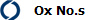Questions 1-4 p31 / 14 p35

• Our understanding of oxidation and reduction was limited to reactions involving oxygen and hydrogen:

2Mg(s)               +               O2(g)          à           2MgO(s)

• Magnesium has been oxidised as it has gained oxygen

• But it has also lost 2 electrons:

Mg(s)               -               2e-                à           Mg2+(s)

• Oxygen has been reduced as it has lost oxygen

• But each oxygen has also gained 2 electrons:

O2(g)               +               4e-                à           2O2-(s)

• A new definition could be used involving electrons and this could be applied to all reactions:

Oxidation                                  - is addition of oxygen / loss of hydrogen /

Is

Loss of electrons

Reduction                                 - loss of oxygen / addition of hydrogen /

Is

Gain of electrons

• Oxidation and reduction must occur simultaneously as all reactions involve a movement of electrons.

• These reactions are given the shorthand term of REDOX reactions.  As they involve

REDuction      and               OXidation

• Redox reactions can now be applied to reaction that do not involve oxygen or hydrogen:

2Fe(s)               +            3Cl2(g)         à           2FeCl3(s)

• Looking at each species often makes it easier to decide which has been oxidised and reduced.

• Adding the oxidation number makes it even easier:

2Fe(s)               +            3Cl2(g)         à           2FeCl3(s)

Fe(s)                                                  à           Fe3+(s)                  +              3e-

0                                                                        +3

• To go from 0 to +3 means electrons have been lost = Oxidation - increases Ox No's

1/2Cl2(g)                +            e-              à           Cl -(s)

0                                                                        -1

• To go from 0 to -1 means that electrons have been gained = Reduction - reduces Ox No's

Oxidising and reducing agents:

2Fe(s)               +            3Cl2(g)         à           2FeCl3(s)

Fe(s)                                                  à           Fe3+(s)                  +              3e-          Oxidation

0                                                                        +3

1/2Cl2(g)                +            2e-              à           Cl -(s)                                               Reduction

0                                                                          -1

• As chlorine accepted the electrons from iron for it to be oxidised we say that chlorine is the oxidising agent

• As iron gave the electrons to chlorine for it to be reduced we say that iron is the reducing agent:

Oxidation                                  Reducing agent

Is

Loss of electrons

Reduction                                 Oxidising agent

Is

Gain of electrons

Examples of oxidising and reducing agents

• Iron ions can exist as Fe2+ and Fe3+:

• Fe2+ solutions are green and Fe3+ solutions are brown.

Fe2+         à   Fe3+        +        e-                Oxidation (requires an oxidising agent)

Green              Brown

• And:

Fe3+         +           e-            à   Fe2+         Reduction (requires a reducing agent)

Brown                                          Green

Using oxidation numbers with equations:

• When assigning oxidation numbers to elements it is a good idea to set it out as below:

 Mg(s) + 2HCl(aq) à MgCl2(aq) + H2(g)

 Mg(s) + 2HCl(aq) à MgCl2(aq) + H2(g) Ox No's 0 +1 -1 +2 -1 0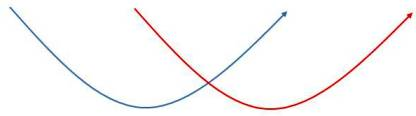0 à+2 +1à 0 (x2) Up 2 Down 2

Things to note:

• The total increase in oxidation numbers by one element is always equal to the total decrease in oxidation numbers by another element.
• Cl- has played no part inn the reaction.  It has not lost / gained electrons.  It has not changed its oxidation number.  These types of ions are called spectator ions.
• Reactions can be rewritten without these spectator ions to make an ionic equation:
 Mg(s) + 2H+(aq) à Mg2+ (aq) + H2(g)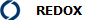Qu 1-2 p33 / Qu 1, 11, 12, 13, 14, 18 P35 / Qu 1, 2a, 5a, 6a, 6b P36, 37